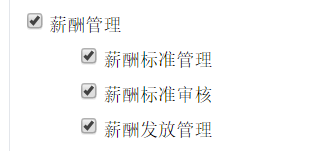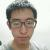## jquery两个方法作用于同一个复选框时，会有一个方法失效

6幽灵9 发布于 2017/03/16 18:54

1.实现父选框改变时子选框的全选与反选2.选择子选矿时，父选框要被自动勾选，而父选框下的子选框都被取消时，父选框自动取消勾选。

代码如下：

``````<script>
function change1(obj, id) {
if (obj.checked) {
\$("#m" + id).attr("checked", true);
} else {
if (\$("[mid=" + id + "]:checked").length == 0) {
\$("#m" + id).attr("checked", false);
}
}
}
function change2(obj2, id2) {
if (obj2.checked) {
\$("[mid=" + id2 + "]").attr("checked", true);
} else {
\$("[mid=" + id2 + "]").attr("checked", false);
}
}
</script>``````
``````<td><c:forEach items="\${menus}" var="m">
<c:if test="\${m.pid==0}">
<input type="checkbox" id="m\${m.id}" name="mids" value="\${m.id}"
onchange="change2(this,\${m.id})" />\${m.mname}<br />
<c:if test="\${m.id==m2.pid}">
&nbsp;&nbsp;&nbsp;<input mid="\${m.id}"
type="checkbox" name="mids" value="\${m2.id}"
onchange="change1(this,\${m.id})" />\${m2.mname}<br />
</c:if>
</c:forEach>
</c:if>
</c:forEach></td>``````

0